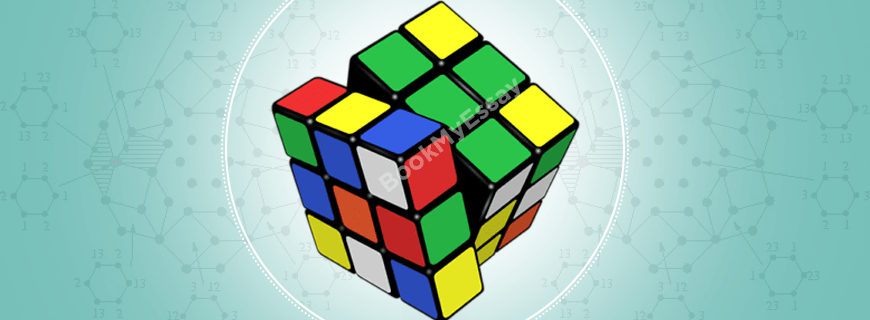# Discrete Mathematics Assignment HelpGet a Free Quote
It's free and always will be.
Page - +
words
NWL

## Discrete Mathematics Assignment Help

Discrete Mathematics is an important part of higher mathematics, which is mostly taught in undergraduate and postgraduate levels. Students of higher mathematics frequently call BookMyEssay for solving assignments they are given in discrete mathematics. The custom assignment writing help service, BookMyEssay, has a huge popularity among the students of mathematics for their top class discrete mathematics assignment help. This assignment help service has a special team of mathematics tutors and experts, who has been handling all sorts of a discrete mathematics assignments, coursework, and homework quite satisfactorily. As per the data available, more than 97% of students, who have availed mathematics assignment help service, have taken help from BookMyEssay once again within 6 months. It is really a clear depiction of authenticity and reliability of BookMyEssay.

### What is Discrete Mathematics?

Discrete Mathematics is not any special branch of mathematics. It is actually a description of a set of different branches of mathematics which are “discrete” in nature, and not “continuous”. It involves discrete elements of mathematics that uses algebra and arithmetic. This branch of mathematics has intensive application in applied mathematics, and computer science. Discrete mathematics provides effective tools for improving mathematical reasoning, interpretation, and problem-solving capabilities of researchers.

The most important members of discrete mathematics include:

• Logic and Boolean algebra
• Graph theory
• Set theory
• Matrix theory
• Hybrid discrete and continuous mathematics
• Relations and functions
• Sequences and series (or “sums“)
• Algorithms and theory of computation
• Number theory
• Induction and recursion
• Counting and discrete probability
• Game theory, decision theory, utility theory, social choice theory
• Discrete analogues of continuous mathematics

To understand discrete mathematics more precisely, its difference with the continuous mathematics need to be understood:

Mathematics can be separated roughly into two sections: the continuous mathematics, and the discrete mathematics. The difference between the two can be nicely demonstrated with the help of wristwatches. Continuous mathematics resembles with analog watches, which has the separate hour, minute, and second hands. The hands move in perfect harmony with each other. In an analog watch, between 10:05PM and 10:06PM, there are substantially many imaginable times as the second-hand sweeps around. In the same way, continuous mathematics is that branch of mathematics that studies all mathematical aspects with the infinite in scope, and where one object can switch over to another object smoothly. As happens in the real number system, between any two real numbers, infinite number of real numbers can be placed. Thus, continuous mathematics provides outstanding models and tools for analyzing real-world incidents that normally change from one phase to another smoothly, e.g. the rhythm of the heart, blood flow, sunrise, movement of a planet etc.

On the contrary, discrete mathematics is similar to a working procedure of a digital watch. One can distinctively mark the difference between any two-specific time, and can watch this incident. A digital watch jumps from one time to the next, and can have different times. Here the transition is sharp and clear-cut. In discrete mathematics, integers play a primary role, unlike the real-number system in continuous mathematics. Discrete mathematics, thus, provides exceptional models and tools for analyzing many real-world incidents that change distinctively, and that lie clearly in a certain state. Discrete mathematics is used in telecommunication, computer science, and genetics, etc.

### How Does BookMyEssay Provide Discrete Mathematics Assignment Writing Help?

Every year, thousands of students get professional discrete mathematics assignment help services from BookMyEssay experts. This branch of mathematics is mainly application based; hence, assignments are mainly given from different fields like computer science, genetics, astronomy, telecommunication, game theory, etc. BookMyEssay provide top class experts to help the students.

The basic characteristics of these discrete mathematics experts include the following:

• They are well qualified people having vast experience in solving complicated practical problems in the different branches of applied mathematics, and using discrete mathematics to solve different categories of real-world problems. So, they can handle any types of discrete mathematics assignments. The departmental head assigns a specific task to the most experienced tutor or expert only.
• BookMyEssay is always very serious regarding the deadline. The expert is given a special reminder from the departmental head regarding the deadline. There is not a single incident of delayed submission has been recorded till to-date.
• The supporting staffs take care of all allied matters to make an assignment presentable.

BookMyEssay has created a fixed mechanism for delivering a completed work within the deadline. In this system, everybody, including the helpdesk executive and assignment writing expert know their sphere of responsibility well. So, there remains no chance of miscommunication with a student, or a delayed submission of an assignment.

### Features of BookMyEssay

• BookMyEssay writers, including Discrete Mathematics assignment help writers always provide 100% plagiarism free job.
• The helpdesk remains active 24/7 throughout the year.
• Whenever required, the experts, and tutors can be accessed for revision, or modification for a completed job.
• On demand, emergency assignment help service can also be availed.
• The service is reasonably priced.